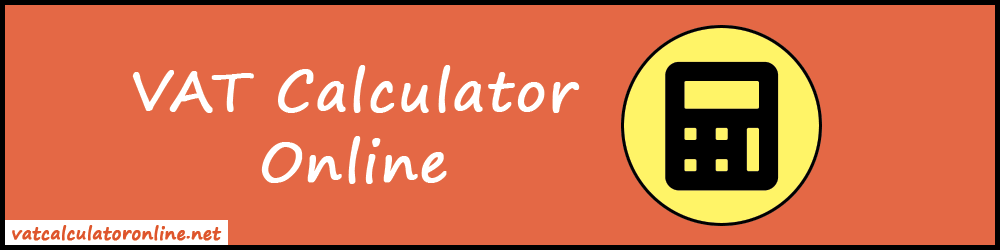# Online VAT Calculator

Use our free online VAT Calculator to add or remove VAT from any amount. It also gives the ability to change the VAT rates.

This VAT Calculator is specially made for UK people. But other country users can also use it to find the VAT inclusive or exclusive rate from the given value. Also, the VAT rates vary for different countries. Therefore, we already have given the feature to change the VAT rates. So, users can change it and calculate the VAT for any country.#### Why we created this tool?

We created this tool in response to recent VAT rate changes. Also, we could not find a similar website on the internet that allows users to adjust the VAT rate. So, we got the idea from there and developed this VAT calculator. It provides business users and individuals with access to change the VAT rate. Even more, they can easily add or deduct the VAT from any Net or Gross figure.

Our tool determines the VAT that businesses charge on goods or services sold in UK. In addition, if the gross value of goods and services subject to VAT is known, it determines the Net price and VAT. In short, you can find VAT inclusive or exclusive both prices in one place.

### How does VAT Calculator Work?

It takes two inputs from the users.

1. Initial Amount (VAT included or excluded)
2. VAT Rate in Percentage

Also, there are two buttons "Add VAT" and "Remove VAT".

• The "Add VAT" button will include the percentage of VAT(%) in the Net price and gives the Gross price and VAT.
• Similarly, the "Remove VAT" button will exclude the percentage of VAT(%) in the Gross price and gives Net price and VAT.

Above all operations are performed in the back-end. Also, it works on pre-defined algorithms that give you 100% accurate and fast results. No need to use the physical calculator. Just keep our tool handy. It will save you time and effort.

### How VAT is Calculated in UK?

There are two operations that we can perform with VAT.

2. Remove VAT

Let's understand both terms in detail with an example.

To calculate the VAT component of the net amount, just multiply the net amount by the VAT rate in percent, divide by 100. Then add the VAT component to the net amount. As a result, you will get the VAT inclusive price at the end.

VAT = Net taxable amount × Applicable VAT rate(%) / 100

##### Example:

Suppose, in the UK, the computer service center charges £160.00 without VAT for a repair. VAT is charged at the standard rate of 20% on the sale. Find the total price with VAT.

##### Solution:

The Net amount is £160.00.

VAT = £160.00 × 20/100 = £32.00

Therefore, the total price including VAT is £160.00 + £32.00 = £192.00

#### 2. Remove VAT

To remove the VAT from any amount, firstly, we will find Net amount by dividing the Gross amount by (1 + VAT percentage) and then subtract it from the Gross amount.

VAT is 20% in the UK, so we will divide the gross amount by 1.20 to get VAT.

Let's take an example to understand it.

##### Example:

Suppose, the VAT inclusive product is £200.00. Find the total Net price excluding VAT.

##### Solution:

Here, the Gross amount is £200.00.

Net Amount = Gross Amount / 1.20 = £200.00 / 1.20 = £166.67

Therefore, the price excluding VAT is £166.67.

Also, you can verify that the above calculations are correct or not by entering the values into the VAT Calculator.

#### How to Use VAT Calculator?

With our online VAT calculator, you can easily add or remove VAT on your invoices with just a few keystrokes. Follow the instructions below to go through the tool.

• Firstly, enter the price that is including or excluding VAT.
• By default, the VAT(%) rate is set to 20%. That is the current standard rate for the United Kingdom. But you can change it if you want.
• To add the VAT, just press the "Add VAT" button. Similarly, to remove the VAT, just press the "Remove VAT" button.
• As a result, it will display the Net Amount, VAT, and Gross Amount.
• Lastly, you can use the "Reset all" button to start the new calculation.

### FAQs

The current standard VAT rate in the UK is 20%.

Yes, Of Course, you can change the VAT rate as you want. That's the main feature of our tool and it makes our tool the most recommended.

No, you don't need to signup or log in to use the calculator. It's an online tool. So, you can just open it in any web browser and start using it.

No, it's completely free for all. No usage limits are set. You can use it as many times as you want. Also, there are no subscription charges to get access.

Yes, you can use it on any small or large device. Because we have made it mobile, iPad, and desktop responsive. So, it works fine on any device.